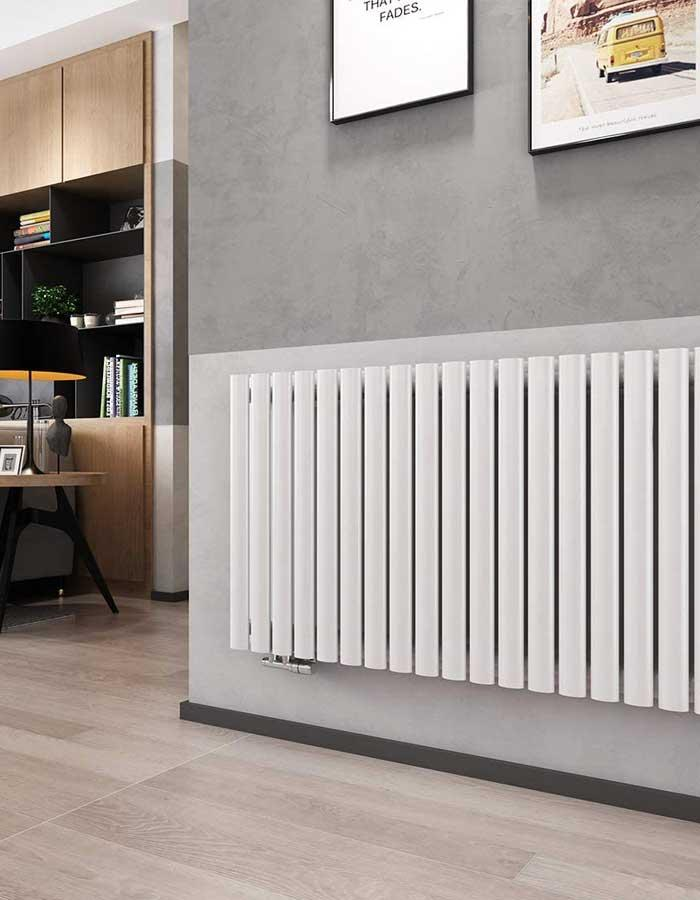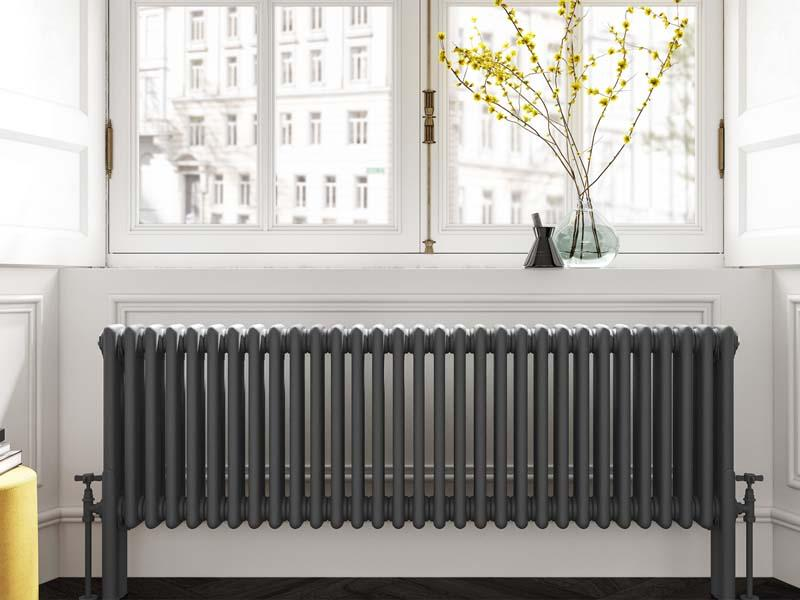#+8613121914007# How to calculate the heat dissipation of column radiator

Click：257 / Publish：2023-05-28 12:57
To calculate the heat dissipation or heat output of a column radiator, you need to consider several factors, including the specific design and dimensions of the radiator. Here's a general approach to estimating the heat dissipation of a column radiator:Determine the Surface Area: Measure the height, width, and depth of the radiator to calculate its total surface area. For a column radiator, multiply the height by the width to find the surface area of each column. Then, multiply the surface area of one column by the total number of columns in the radiator to get the overall surface area.

Determine the heat output per Square Meter: The heat output of a radiator is typically measured in watts per square meter (W/m²) or British Thermal Units per hour per square foot (BTU/hr/ft²). This value represents the amount of heat the radiator can emit per unit of surface area. Consult the radiator manufacturer's specifications or technical data to find the heat output per square meter or per square foot.

Calculate the Total heat output: Multiply the surface area of the radiator (in square meters or square feet) by the heat output per square meter or per square foot. This calculation will give you the estimated heat dissipation or heat output of the column radiator.

It's important to note that this method provides an estimation and the actual heat dissipation of a column radiator may vary based on other factors, such as the operating conditions, water temperature, and the efficiency of the heating system. For precise calculations and to ensure accurate heat output, it is recommended to consult the manufacturer's guidelines or seek professional advice.